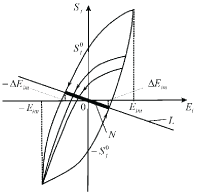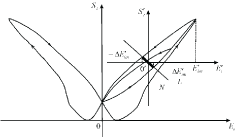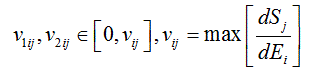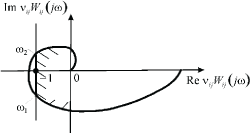## Absolute Stability of Control System with Electromagnet Elastic Actuator for Nanotechnology and Nanoscience

Afonin SM1*

1 National Research University of Electronic Technology MIET, Moscow, Russia.

*Corresponding Author: Afonin SM, National Research University of Electronic Technology MIET, Moscow, Russia, TEL: +74997314441 ; FAX: +74997314441;E-mail:learner01@mail.ru

Citation: Afonin SM (2019) Absolute Stability of Control System with Electromagnet Elastic Actuator for Nanotechnology and Nanoscience. Nano Technol & Nano Sci J 2:111.

Copyright: © 2019 Afonin SM, et al. This is an open-access article distributed under the terms of the Creative Commons Attribution License, which permits unrestricted use, distribution, and reproduction in any medium, provided the original author and source are credited

Received date: Sep 26, 2019; Accepted date: Oct 14, 2019; Published date: Oct 17, 2019.

## Abstract

In this paper, we receive the stationary set of the control system of the hysteresis and butterfly deformation of the electromagneto elastic actuator in form the segment of the straight line. The aim of this paper is to determine the condition of the absolute stability on the derivative for control system of the deformation of the electromagnetoelastic actuator for nanotechnology and nanoscience. We used the frequency methods for Lyapunov stable control system to calculate the absolute stability of the control system with electromagnetoelastic actuator for nanotechnology and nanoscience.

## Keywords

Condition Absolute Stability, Control System, Deformation, Electromagnetoelastic Actuator, Hysteresis Characteristics, Butterfly Characteristic, Stationary Set, Piezoactuator, Transfer F Unction.

## Introducton

In this paper, we determine the condition of the absolute stability on the derivative for control system of the deformation of the electromagnetoelastic actuator. The control systems with electromagnetoelastic actuator on piezoelectric, electrostrictive and magnetostrictive effects solves problems of the precise matching in the nanotechnology and nanoscience, the compensation of the temperature and gravitational deformations of the equipment, the wave front correction in the adaptive laser system [1-14]. The piezoactuator for nanotechnology and nanoscience is used in the scanning tunneling microscope, the scanning force microscope, the atomic force microscope, in the gene manipulator [15-30].

The problems of using criteria absolute stability of the control system with electromagnetoelastic actuator for nanotechnology and nanoscience are discussed. The stationary set of the control system of the deformation of the electromagnetoelastic actuator is received.

## Absolute Stability Control System with Electromagnetoelastic Actuator

The electromagnetoelastic actuator on piezoelectric, electrostrictive or magnetostrictive effects is used in the control systems for the micro and nano surgical repairs, the micro and nano robotics, the micro and nano manipulators and injectors for nanotechnology and nanoscience [1-6]. The aim of this work is to calculate the condition of the absolute stability on the derivative for control system of the deformation of the electromagnetoelastic actuator for nanotechnology and nanoscience.

The frequency methods for Lyapunov stable control system are used to determine the condition of the absolute stability of control system  with electromagnetoelastic actuator for nanotechnology and nanoscience.

We receive the sufficient absolute stability condition of the control system with the hysteresis nonlinearity of the electromagnetoelastic actuator using the Yakubovich absolute stability criterion with the condition on the derivative. This criterion is the development of the Popov absolute stability criterion . For the Lyapunov stable control system and the Yalubovich absolute stability criterion for the systems with the single hysteresis nonlinearity provides the simplest and pictorial representation of results of the investigation of the stability of the strain control system with the electromagnetoelastic actuator for nanotechnology and nanoscience.

For description of the control system we use the transfer function of the linear part of the system Wij (P) and the hysteresis function of the relative deformation Sj of the electromagnetoelastic actuator . We have the hysteresis characteristic of the electromagnetoelastic actuator in the following formWe receive the stationary set for the deformation of the piezoactuator on Figure 1 for the stable linear part of the control system, where the equation for the straight-line L has the form

Ei + Wij(0)Sj = 0

In static regime for the control system for the deformation of the piezoactuator we receive the value of the transfer function Wij (0) of the linear part of the control system. The set of points N for intersection of this straight line L with the hysteresis characteristic represents the segment of the straight line marked on Figure 1. At Ei0 = Ei(0) and Sj0 = Sj (0) we have the stationary solution to the control system with hysteresis.

We obtain the stationary set N of the system in the form of the marked segment of straight line L in Figure 1 with the set of pairs (Ei0, Sj0). Each point of intersection of the hysteresis nonlinearity with the partial loops and the straight-line L corresponds to one equilibrium position with the coordinates (Ei0, Sj0). Therefore, for the stationary set the equation has the following form

Ei0 + Wij(0)Sj0 = 0.

Let us consider butterfly characteristic of the deformation of the electromagnetoelastic actuator for nanotechnology and nanoscience. For the actuator with the electro strictive effect the deformation characteristic on butterfly wings is observed for unipolar change of the electric field strength on Figure 2. We have the particular cycle on one wing of butterfly in the form of the hysteresis loop. For butterfly type characteristic deformation of actuator in the control system the coordinate origin is moved to new zero with top dash on Figure 2.

For hysteresis loop on butterfly type characteristic we have stationary set N of the system marked segment of straight line L in Figure 2. For the magnetostrictive actuator the deformation characteristic has the butterfly type.

We receive the continuous function Sj(Ei) the hysteresis loop of the piezoactuator and the quantities of the derivativeFigure 2: Butterfly characteristic deformation of actuator with electro strictive effectwhere the quantities of the derivative V1ij and V2ij are calculated using the hysteresis characteristic on Figure 1 for the maximum admissible electric field strength in the piezoactuator. The quantities V1ij = 0 and V2ij = Vij are the minimum and the maximum values of the tangent of the inclination angle of the tangent line to the hysteresis nonlinearity of the piezoactuator. Thus, we obtain

v33: v31: v15 = d33: d31: d15,

where the ratios of the tangents of the inclination angle of the tangent line to the hysteresis nonlinearity of the piezoactuator for longitudinal, transverse and shift piezoeffects are proportional to the ratios of the piezomodules. We have the expression for the sufficient absolute stability condition of the system with the hysteresis nonlinearity of the electromagnetoelastic actuator using the Yakubovich absolute stability criterion with the condition on the derivative. The Yalubovich criterion is the development of the Popov absolute stability criterion .

For the Lyapunov stabile control system the Yalubovich absolute stability criterion for the system with the single hysteresis nonlinearity provides the simplest and pictorial representation of results of the investigation of the stability control system. The sufficient condition of the absolute stability the control system for the deformation of the electromagnetoelastic actuator at V1ij = 0 and V2ij = Vij have the form

ReVijWij(jω) ≥ -1,

where VijWij(jω)is the amplitude-phase characteristic of the open-loop system and in brackets j is the imaginary unity and ω is the frequency.

The amplitude-phase characteristic of the open-loop system VijWij(jω) on Figure 3 should be situated to the right of the straight lineReVijWij(jω) = -1,

for all values of ω ≥ 0.

On the plane of the logarithmic amplitude frequency characteristic and the phase frequency characteristic in the form L(ω) = Q[Ï•(ω)],L(ω) = 20lgi|VijWij(jω)| we have for the absolute stability criterion the control system with electromagnetoelastic actuator the corrected logarithmic amplitude frequency characteristic below the boundary curve in the form

L(ω) = 20 lg|1/ cos Ï•|.

For the piezoactuator from PZT we received the value of the maximum tangent of the inclination angle of the tangent line to hysteresis nonlinearity about 1 nm/V for longitudinal piezoeffect and 0.6 nm/V for transverse piezoeffect.

## Results and Discussion

For hysteresis and butterfly characteristic in control systems the stationary set represents the segment of the straight line bounded by hysteresis loop.

The frequency methods are used for Lyapunov stable control system to calculate the condition the absolute stability of the control system with electromagnetoelastic actuator for nanotechnology and nanoscience.

## Conclusions

We determined the stationary set of the control system of the deformation of the electromagnetoelastic actuator. The stationary set of the system is the segment of straight line bounded by hysteresis loop for hysteresis and butterfly characteristic deformation of the actuator.

We received condition of the absolute stability on the derivative for the control system with the electro magneto elastic actuator for nanotechnology and nanoscience.